## The Classical Adjoint of a Square Matrix

Let A = [ a ij ] be a square matrix. The transpose of the matrix whose ( i, j) entry is the a ij cofactor is called the classical adjoint of A: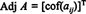Example 1: Find the adjoint of the matrixThe first step is to evaluate the cofactor of every entry:Therefore,Why form the adjoint matrix? First, verify the following calculation where the matrix A above is multiplied by its adjoint:Now, since a Laplace expansion by the first column of A givesequation (*) becomes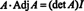This result gives the following equation for the inverse of A: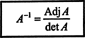By generalizing these calculations to an arbitrary n by n matrix, the following theorem can be proved:

Theorem H. A square matrix A is invertible if and only if its determinant is not zero, and its inverse is obtained by multiplying the adjoint of A by (det A) −1. [Note: A matrix whose determinant is 0 is said to be singular; therefore, a matrix is invertible if and only if it is nonsingular.]

Example 2: Determine the inverse of the following matrix by first computing its adjoint:First, evaluate the cofactor of each entry in A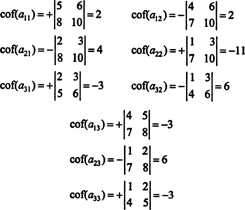These computations imply that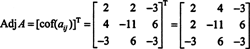Now, since Laplace expansion along the first row gives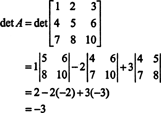the inverse of A is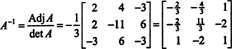which may be verified by checking that AA −1 = A −1 A = I.

Example 3: If A is an invertible n by n matrix, compute the determinant of Adj A in terms of det A.

Because A is invertible, the equation A −1 = Adj A/det A impliesRecall that if B is n x n and k is a scalar, then det( kB) = k n det B. Applying this formula with k = det A and B = A −1 gives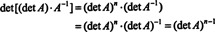Thus,Example 4: Show that the adjoint of the adjoint of A is guaranteed to equal A if A is an invertible 2 by 2 matrix, but not if A is an invertible square matrix of higher order.

First, the equation A · Adj A = (det A) I can be rewritten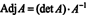which impliesNext, the equation A · Adj A = (det A) I also implies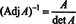This expression, along with the result of Example 3, transforms (*) intowhere n is the size of the square matrix A. If n = 2, then (det A) n−2 = (det A) 0 = 1—since det A ≠ 0—which implies Adj (Adj A) = A, as desired. However, if n > 2, then (det A) n−2 will not equal 1 for every nonzero value of det A, so Adj (Adj A) will not necessarily equal A. Yet this proof does show that whatever the size of the matrix, Adj (Adj A) will equal A if det A = 1.

Example 5: Consider the vector space C 2( a, b) of functions which have a continuous second derivative on the interval ( a, b) ⊂ R. If f, g, and h are functions in this space, then the following determinant,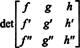is called the Wronskian of f, g, and h. What does the value of the Wronskian say about the linear independence of the functions f, g, and h?

The functions f, g, and h are linearly independent if the only scalars c 1, c 2, and c 3 which satisfy the equation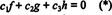are c 1 = c 2 = c 3 = 0. One way to obtain three equations to solve for the three unknowns c 1, c 2, and c 3 is to differentiate (*) and then to differentiate it again. The result is the system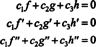which can be written in matrix form aswhere c = ( c 1, c 2, c 3) T. A homogeneous square system—such as this one—has only the trivial solution if and only if the determinant of the coefficient matrix is nonzero. But if c = 0 is the only solution to (**), then c 1 = c 2 = c 3 = 0 is the only solution to (*), and the functions f, g, and h are linearly independent. Therefore,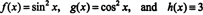To illustrate this result, consider the functions f, g, and h defined by the equations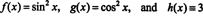Since the Wronskian of these functions isthese functions are linearly dependent.

Here's another illustration. Consider the functions f, g, and h in the space C 2(1/2, ∞) defined by the equations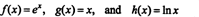By a Laplace expansion along the second column, the Wronskian of these functions is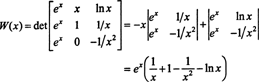Since this function is not identically zero on the interval (1/2, ∞)—for example, when x = 1, W( x) = W(1) = e ≠ 0—the functions f, g, and h are linearly independent.